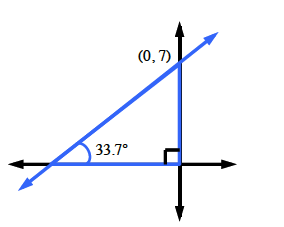### Home > GC > Chapter 5 > Lesson 5.1.4 > Problem5-37

5-37.

Find the equation of the line that has a $33.7^\circ$ slope angle and a $y$-intercept at $(0,7)$. Assume the line has a positive slope.

Sketch a graph and a slope triangle for the line.$Δx=?$
$Δy=7$

Use the tangent ratio to find the slope:

$\frac{\Delta y}{\Delta x}=\text{slope} = \text{tan}(\theta)$

Once you know the slope and the $y$-intercept, you can write the equation of the line. You use slope-intercept form $(y=mx+b)$.

$y \approx \frac{2}{3}x + 7$